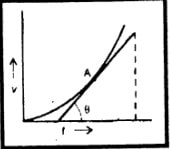Sketch a velocity-time graph for the motion of the body. From the graph explaining each step, calculate the total distance covered by the body.

Difficulty: Medium

Velocity time graph:

Calculation of distance moved by an object from a velocity-time graph:

The distance moved by an object can also be determined by using its velocity-time graph.

1. If an object moves at constant velocity v for time t. The distance covered by the object is v x t. the distance can also be found by calculating the area under the velocity-time graph. This area is shaded and is equal to v x t.

2. If the velocity of the object increases uniformly from 0 to v in time t. the magnitude of its average.

avg. velocity = $\frac{0 \: + v}{2}$

=$\frac{v}{2}$

$Distance \: covered = average \: velocity\times \: time =\frac{1}{2v}\times \:t$

Now we calculate the area under the velocity-time graph, which is equal to the area of the triangle shaded in the figure.

Its \: value \: is \: equal \: to \: $\frac{1}{2} \: base\times \: height =\frac{1}{2} \: v\times t$

Note:

The area between the velocity-time graph and the time axis is numerically equal to the distance covered by the object.